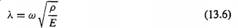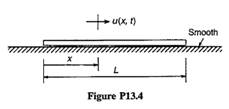### Create an Account

Home / Questions / The axial frequencies of a free-free structure are sometimes of great importance as in the...

# The axial frequencies of a free-free structure are sometimes of great importance as in the “Pogo” stability problem experienced by some rockets Consider axial motion of the uniform free-free bar in

The axial frequencies of a free-free structure are sometimes of great importance, as in the “Pogo” stability problem experienced by some rockets. Consider axial motion of the uniform free-free bar in Fig. P13.4. (a) Show that the system has a zero-frequency, or rigidbody, mode, (b) Obtain the characteristic equation from which the nonzero eigenvalues can be determined. Your answer should contain the parameters a and L, where a is defined by Eq. 13.6. (c) Determine an expression for the fundamental (flexible) axial frequency of the bar (coi rad/s), (d) Determine an expression for the fundamental (flexible) axial mode shape, 0i(x), and use M a t l a b , or another computer program, to plot the fundamental mode. Scale the mode so that its maximum value is 1.0.May 19 2020 View more View LessSubscribe To Get Solution